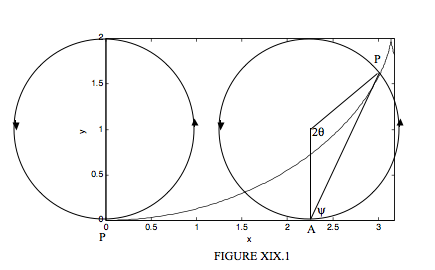$$\require{cancel}$$

# 19.1: Introduction to Cycloids

•• Contributed by Jeremy Tatum
• Emeritus Professor (Physics & Astronomy) at University of Victoria

Let us set up a coordinate system $$Oxy$$, and a horizontal straight line $$y = 2a$$. We imagine a circle of diameter $$2a$$ between the $$x$$-axis and the line $$y = 2a$$, and initially the lowest point on the circle, P, coincides with the origin of coordinates O. We now allow the circle to roll counterclockwise without slipping on the line $$y = 2a$$, so that the centre of the circle moves to the right. As the circle rolls on the line, the point P describes a curve, which is known as a cycloid.When the circle has rolled through an angle $$2\theta$$, the centre of the circle has moved to the right by a horizontal distance $$2a \theta$$, while the horizontal distance of the point P from the centre of the circle is $$a \sin 2 \theta$$ and the vertical distance of the point $$P$$ below the centre of the circle is $$a \cos 2 \theta$$. Thus the coordinates of the point $$P$$ are

$x = a(2 \theta + \sin 2 \theta) \label{19.1.1} \tag{19.1.1}$

and

$y = a (1 - \cos 2 \theta ). \label{19.1.2}\tag{19.1.2}$

Equations $$\ref{19.1.1}$$ and $$\ref{19.1.2}$$ are the parametric equations of the cycloid. Using a simple trigonometric identity, Equation $$\ref{19.1.2}$$ can also be written

$y = 2a \sin^2 \theta . \label{19.1.3}\tag{19.1.3}$

Example $$\PageIndex{1}$$

When the $$x$$-coordinate of P is 2.500$$a$$, what (to four significant figures) is its $$y$$-coordinate?

Solution

We have to find $$2 \theta$$ by solution of $$2 \theta +\sin 2 \theta$$. By Newton-Raphson iteration or otherwise, we find $$2 \theta$$ = 0.931 599 201 radians, and hence y = 0.9316$$a$$.